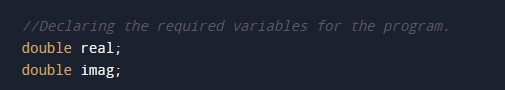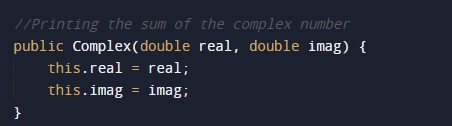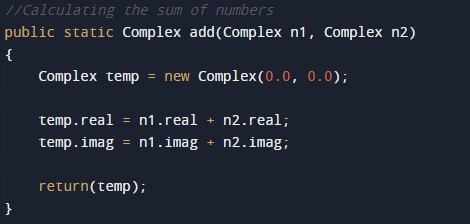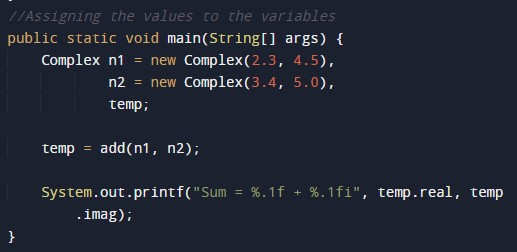# Java Program to add two complex numbers

In this tutorial you will learn about the Java Program to add two complex numbers and its application with practical example.

## Java Program to add two complex numbers

In this tutorial, we will learn to create a Java program that will add two Complex Numbers using Java programming.

## Prerequisites.

Before starting with this tutorial, we assume that you are the best aware of the following java programming topics:

• Operators in Java Programming.
• Basic Input and Output function in Java Programming.
• Basic Java programming.
• While loop in Java programming.

## What is a Complex Number?

A number is said to be a complex number if it is in the form of x+yi, where x and are real numbers, and i is an indeterminate satisfying the condition i2 = −1.

For example, 2 + 3i

## Program to add two Complex Numbers:-

In this program, we will first declare the values of the variables. Then we will add two numbers using the functions in java. At last, we will print the output of the program to the user using a print function.

## Output:-In the above program, we have first initialized the required variable.• temp = it will hold the complex value.
• real = it will hold the float value.
• img = it will hold the integer value.

Taking the input integer number and exponent from the user.Calculating the complex numbers.Printing the output numbers.In this tutorial we have learn about the Java Program to add two complex numbers and its application with practical example. I hope you will like this tutorial.